# Portfolios of Two Assets

## Characteristics of a Two-asset Portfolio

The formulas and MATLAB functions discussed previously are sufficient to compute the characteristics of any portfolio. However, to better understand the economics of portfolio construction it is useful to consider the effects of combining two assets to form a portfolio.

To economize on notation we omit parentheses. Thus x1 and x2 will be the proportions invested in assets 1 and 2 respectively, and e1 and e2 will be their expected returns. The expected return of the portfolio, ep, will then be:

```
ep = x1*e1 + x2*e2
```

Since the proportions will sum to 1.0 we may also write:

```
ep = e1 + x2*(e2-e1)
```

The variance of the portfolio, vp, will be a function of the proportions invested in the assets, their return variances (v1 and v2), and the covariance between their returns (c12):

```
vp = ((x1^2)*v1) + (x2^2)*v2) + 2*x1*x2*c12
```

Here too, we can substitute (1-x2) for x1 to obtain an expression relating the portfolio's variance to the amount invested in asset 2:

```
vp = v1 + 2*x2*(c12-v1) + (x2^2)*(v1-2*c12+v2)
```

The standard deviation of return (sp) will, as always, be the square root of the variance:

```
sp = sqrt(vp)
```

Throughout this section we will assume that asset 1 has less risk (v1&ltv2) and a smaller expected return (e1&lte2). We will be interested in the risk-return tradeoff associated with different combinations of the two assets and, in particular, the shape of the curves in mean-variance and mean-standard deviation space that result as more money is invested in the risky asset (that is, as x2 is increased and x1 decreased).

## Combining a Riskless Asset with a Risky Asset

The figure below plots the locus of mean-standard deviation combinations for values of x2 between 0 and 1 when e1=6, e2=10, s1=0, s2=15 and c12=0.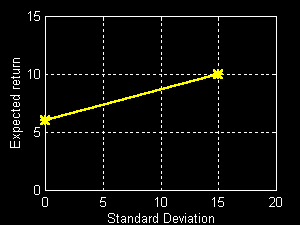In this case, as in every case involving a riskless and a risky asset, the relationship is linear. This is easily seen. Recall that ep is always linear in x2 as shown earlier. If asset 1 is riskless, sp will also be linear in x2, since both v1 and c12 will equal zero. In such a case:

```
vp = (x2^2)*v2
sp = sqrt((x2^2)*v2)
= abs(x2)*sqrt(v2)
= abs(x2)*s2
```

where abs(x2) denotes the absolute value of x2.

This result can be extended to cases in which it is possible to take short positions. First, assume one can either go long the riskless asset ('lend") or take a short position in it ("borrow") at the same interest rate (e1). The formulas above then apply directly. The figure below shows the results obtained by using leverage in this manner. For example, the point marked 1.5 is associated with x2=1.5 and x1=-0.5. It indicates that by "levering up" an investment in asset 2 by 50% an Investor can obtain a probability distribution of return on initial capital with an expected value of 12% and a standard deviation of 22.5%. The other points in the figure correspond to the indicated values of x2. Those above the original point involve borrowing (x1<0) while those below it involve lending (x1>0).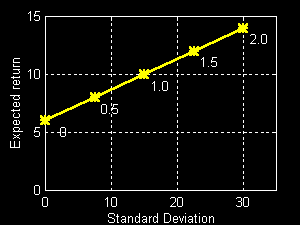What if an Investor could short the risky security (x2<0) and invest the proceeds obtained from the short sale in the riskless security? Here too, the standard formulas apply. However, note that the variance will be positive, as will the standard deviation, since a negative number (x2) squared is always positive. The figure below shows the effects of negative x2 values.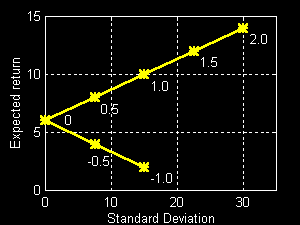A qualification to this analysis is in order. A strategy involving short positions may require that additional capital be pledged to cover possible shortfalls between ending asset and liability values. Absent this, a higher rate will typically be charged for the short position so the income to the lender in states of the world in which the borrower is solvent will be sufficiently high to compensate for the shortfalls associated with the states of the world in which the borrower is insolvent.

The figure below shows a simple case of this sort in which funds may be borrowed, but at a higher rate (8%) than the rate at which they may be lent (6%). Here the locus of the ep,sp combinations plots as two lines, the first associated with the lower lending rate, the second with the higher borrowing rate. As before, the risky asset offers an expected return of 10% and a risk of 15%. The efficient frontier is shown by the solid lines. The dashed line indicates the options that would be available if the Investor could lend at 8%. While points on it are infeasible, those plotting on its extension to the right of the point representing the risky asset are both feasible and efficient.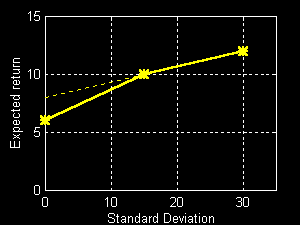In practice the rate charged for borrowing may increase with the amount borrowed. In such a case the locus of ep,sp combinations will increase at a decreasing rate as risk (sp) increases beyond the amount associated with a full unlevered investment in the risky asset (x2=1). Under these conditions there will eventually be decreasing returns to risk-taking.

## Combining Two Risky Assets

When a portfolio includes two risky assets, the Analyst needs to take into account expected returns, variances and the covariance (or correlation) between the assets' returns. The differences from the earlier case in which one asset is riskless occur in the formula for portfolio variance. In terms of risks and correlations it is:

```
vp = ((x1^2)*(s1^2)) + (2*x1*x2*r12*s1*s2) + ((x2^2)*(s2^2))
```

where r12 is the correlation between the assets' returns.

## Combining Two Perfectly Positively Correlated Risky Assets

To begin, consider the case in which two returns are perfectly positively correlated. Under these conditions:

```
vp = ((x1^2)*(s1^2)) + (2*x1*x2*s1*s2) + ((x2^2)*(s2^2))
```

The term on the right can be factored to obtain:

```
vp = (x1*s1 + x2*s2)^2
```

from which it follows that:

```
sp = abs(x1*s1 + x2*s2)
```

where, abs(..) connotes the absolute value of the enclosed expression. As long as x1 and x2 are both non-negative the expression itself will be non-negative since neither s1 nor s2 can ever be negative. However, if one of the two x values is sufficiently negative, the absolute value must be utilized explicitly.

Consider combinations of long positions in the two assets (x1>=0, x2>=0). For any such combination:

```
sp = x1*s1 + x2*s2
```

And, as always:

```
ep = x1*e1 + x2*e2
```

In such a case both risk and return will be proportional to x2:

```
sp = s1 + x2*(s2-s1)
ep = e1 + x2*(e2-e1)
```

and all such portfolios will plot on a straight line connecting the points representing the two assets. In the figure below, e1=8,s1=5, e2=10, s2=15 and r12=1.0.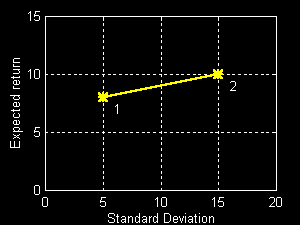This relationship can be extended by allowing x2 to be either greater than one or less than zero. Of particular interest is the combination that gives the smallest possible risk: the minimum-variance portfolio. In this case it is possible to achieve a variance of zero! We seek a value x2 for which:

```
sp = s1 + x2*(s2-s1) = 0
```

This will be obtained when:

```
x2 = -s1/(s2-s1)
```

and

```
x1 = 1-x2
= 1 + (s1/(s1+s2)
= s2/(s2-s1)
```

In our example a riskless portfolio can be obtained by setting:

```
x2 = -5/(15-5) = -0.5
x1 = 1-x2 = 1.5
```

This can be accomplished by taking a short position in asset 2 equal to one-half the Investor's funds and investing the proceeds as well as the original amount of money in asset 1. In practice this may require the pledging of some other collateral to provide a sufficient guarantee to the lender of asset 2 that the short position can be covered when needed.

The ability to form a riskless portfolio by taking offsetting positions in two perfectly positively correlated assets leads directly to a figure similar to that derived earlier when a riskless asset was combined with a risky one. Let the expected return on the zero-variance portfolio be:

```
e0 = e1 + x2min(*e2-e1)
```

where:

```
x2min = -s1/(s2-s1)
```

In the current example e0 will equal 7% (8-0.5*(10-8)). The set of portfolio risks and returns can then be derived by considering combinations of this riskless asset (portfolio) and either asset 1 or asset 2. Either view will provide the familiar graph associated with risky and a riskless asset. In this case: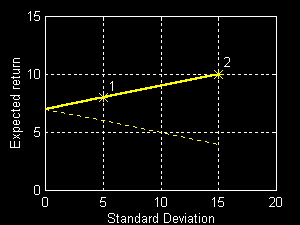While all the combinations shown are feasible, only those on the upper line are efficient -- a point emphasized by the use of a dashed line for the dominated portion of the relationship.

## Combining Imperfectly Correlated Risky Assets

While perfectly positively correlated risky assets do exist, they are the exception rather than the rule. In most cases correlation coefficients are less than 1.0. The implications of this fact for risk are central to an understanding of the effects of diversification.

Consider a portfolio with long positions in two risky assets (x1>0, x2>0). As shown earlier, its variance will be:

```
vp = ((x1^2)*(s1^2)) + (2*x1*x2*r12*s1*s2) + ((x2^2)*(s2^2))
```

Now imagine two cases, similar in every respect (x1,x2,e1,e2,s1,s2) but correlation (r12). Let vp(1) be the variance of one portfolio, for which r12=1 and vp(r) be the variance of the other, for which r12=r, where r is less than 1. Only the middle term in the equation for portfolio variance will differ in the two calculations. Since all the components of that term but r12 are positive, it follows that vp(r)&ltvp(1). More generally, other things equal:

```
vp(r1) 0, x2>0
```

Other things equal, the smaller the correlation between two assets, the smaller will be the risk of a portfolio of long positions in the two assets.

The figure below shows combinations of risk and return for such portfolios when e1=8,s1=5, e2=10 and s2=15. Each curve applies to a case with a different correlation between the two assets' returns. Not surprisingly, the cases are coincident at the end-points (x1=1,x2=0 and x1=0,x2=1). For all interior combinations, when the correlation coefficient is less than 1.0, risk is less than proportional to the risks of the two assets, with the extent of risk reduction greater, the smaller the correlation coefficient. Thus the yellow curve (r12=1.0) provides no risk reduction, only risk-averaging; the red curve (r12=0.5) provides some risk reduction, the green curve (r12=0) more, and the blue curve (r12=-0.5) even more.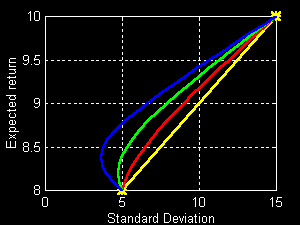The most powerful case of diversification arises when r12=-1.0. In this instance:

```
vp = ((x1^2)*(s1^2)) - (2*x1*x2*s1*s2) + ((x2^2)*(s2^2))
```

which can be factored to obtain:

```
vp = (x1*s1 - x2*s2)^2
```

and

```
sp = abs(x1*s1 - x2*s2)
```

In such a case the minimum-variance portfolio will be riskless. To obtain it, we wish to set:

```
x1*s1 - x2*s2 = 0
given: x1+x2 = 1
```

The solution is:

```
x2 = s1/(s1+s2)
```

Thus if s1=5 and s2=15 and the two assets are perfectly negatively correlated, a riskless portfolio will be obtained with x2=5/(5+15)=0.25 and x1=0.75. Its expected return will equal 0.25*e1+0.75*e2 (here, 8.5%). The next figure repeats the results of the prior case and adds this new one (in white).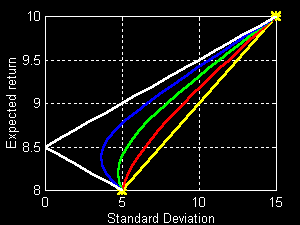Once again we have obtained the familiar diagram in which there is a riskless asset. This should not be a surprise. Long positions in two perfectly negatively correlated assets are similar to (1) a long position in one of two perfectly positively correlated assets and (2) a short position in the other.

In most cases asset correlations lie between -1.0 and +1.0. To cover all possibilities we need a general formula for the minimum-variance portfolio. For this we start with a reduced-form equation in which vp is expressed as a function of x2 (under the assumption that x1=1-x2):

```
vp = v1 + x2*(c12-v1) + (x2^2)*(v1-2*c12+v2)
```

The derivative with respect to x2 is:

```
d(vp)/d(x2) = (c12-v1) + 2*x2*(v1-2*c12+v2)
```

Setting this to zero gives:

```
x2min = (v1-c12)/(2*(v1-2*c12+v2))
```

The minimum-variance portfolio may have a lower risk than either of its component assets. It may also have a higher return. Consider the point at which x2=0. Here:

```
d(ep)/d(x2) = e2-e1
d(vp)/d(x2) = c12-v1
```

and:

```
d(ep)/d(vp) = (e2-e1)/(c12-v1)
```

As usual, we assume that e2>e1. For the slope d(ep)/d(vp) to be negative we need:

```
c12-v1 <0 ```

That is:

```
r12*s1*s2
```

or:

```    r12
```

For example, if s1=5, s2=15, e2>e1 and r12<5/15, the minimum variance portfolio will dominate asset 1, offering both lower risk and higher expected return.

The figure below shows a case in which e1=8,s1=5, e2=10,s2=15 and r12=0.10.  Here, the risk-return plot "bends backward" so that x1=1 is an inefficient portfolio.   The  minimum-variance portfolio is efficient, as are portfolios that combine it (in non-negative amounts) with  asset 2.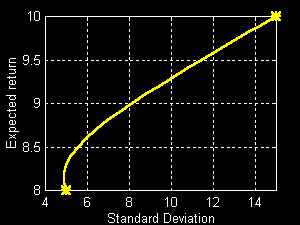If r12 exceeds s1/s2, the minimum variance portfolio will require a short position in asset 1. The figure below shows a case in which e1=8,s1=5, e2=10,s2=15 and r12=0.80.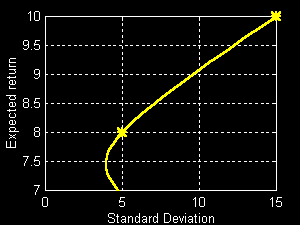As before, all points above and to the right of the point representing the minimum-variance portfolio are efficient. In this case, asset 1 is efficient, as are all combinations involving asset 2 in amounts exceeding x2min. Note that the efficient frontier increases at a decreasing rate, exhibiting decreasing returns to risk- bearing.

## Risk-return Tradeoffs in Mean-Variance Space

We have seen that efficient combinations of two assets plot on a curve in mean-standard deviation space  that increases at either a constant rate or at a decreasing rate as standard deviation is increased. What can  be said about the shape of the frontier in mean-variance space?

First, note that:

```
vp = sp^2
```
```Thus:
```
```
d(vp) = 2*sp*d(sp)
```
```and:
```
```
d(sp) = d(vp)/(2*sp)
```

From this it follows that:

```
d(ep)/d(vp) = (d(ep)/d(sp))/(2*sp)
```

If d(ep)/d(sp) is constant as sp is increased, then d(ep)/d(vp)  will be a decreasing function of sp.  The figures below provide illustrations of the efficient frontiers in each of the two spaces when e1=6, s1=0,  e2=10, s2=15 and the two assets are perfectly positively correlated..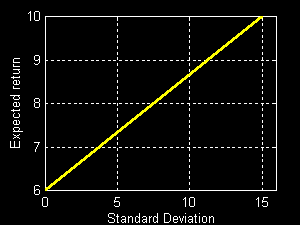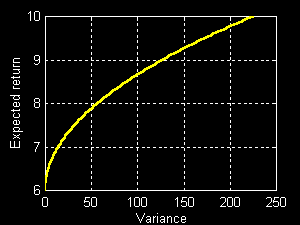If two risky assets are less than perfectly correlated, d(ep)/d(sp) will decrease with sp and d(ep)/d(vp) will, in a sense, decrease at an even faster rate. The figures below provide illustrations of the frontier representing non-negative combinations of the two assets in each of the two spaces when e1=6,s1=5, e2=10, s2=15 and r12=0.5.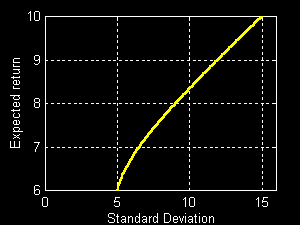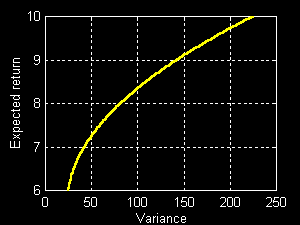When two assets are combined to form portfolios, the efficient frontier will plot as a curve with a decreasing slope in mean-variance space, no matter what the assets' characteristics. This implies that there will be a unique point of tangency with an indifference curve (line) from a family exhibiting constant risk tolerance (that is, for which utility = ep-vp/t).

The figures below provide illustrations using the assets in the most recent example and a risk tolerance of 50. In each diagram the optimal portfolio lies at the point at which the green indifference curve is tangent to the efficient frontier. Of course the optimal portfolio is the same in each diagram. In this case the optimal mix has x1=0.5 and x2 =0.5. The expected return of the portfolio is 8.0%, its variance is 81.25, its standard deviation 9.0139 and it provides the Investor in question a utility of 6.375%. The latter can be seen by inspecting the vertical intercept of the green indifference curve. The points on the blue indifference curve provide a lower level of utility and thus are inappropriate for this Investor. Points on the red indifference curve provide a higher level of utility, but none is feasible in this case.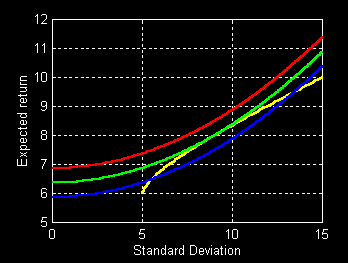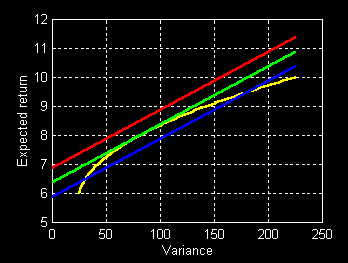## The Excess Return Sharpe Ratio

A widely-used (and sometimes misused) measure of investment performance is the Sharpe Ratio, originally named the reward-to-variability ratio by its author, but now  commonly given this eponymous description.  Broadly defined, it is the ratio of the expected value of a  zero-investment strategy to the standard deviation of that strategy. An important special case involves a  zero-investment strategy in which funds are borrowed at a fixed rate and invested in a risky asset.

Let x be invested in asset 2 and -x in asset 1. In our previous notation, this is equivalent to x2=x and x1=- x. The expected return relative to the underlying or notional value x will be:

```
e = x*(e2-e1)
```
```while the standard deviation will be:
```
```
s = x*s2
```
```The Sharpe Ratio for the strategy will thus be:
```
```
e/s = (x*(e2-e1))/(x*s2)
```
```or:
```
```
(e2-e1)/s2
```

Note that e2-e1 is the expected value of the difference between the return on asset 2 and the return on asset 1.  The difference between the return of a risky asset and that of a riskless one is termed the risky  asset's excess return:

```
xr2 = r2-r1
```

Since r1 is riskless, the standard deviation of xr2 will equal the standard deviation of r2.  Thus the Sharpe Ratio for our strategy will be:

```
e(xr2)/s(xr2)
```

that is, the expected excess return divided by the standard deviation of excess return.   For specificity we  will call this asset 2's excess return Sharpe Ratio (xrsr), although it is often termed simply  the asset's Sharpe Ratio.

Note that x, the scale of the zero-investment strategy, does not appear in the formula -- all strategies involving a given asset or portfolio have the same value of xrsr, no matter what their scale (assuming, of course, that the rate of interest is unaffected by the amount borrowed). Under these conditions, xrsr is scale-independent.

To see one of the implications of this characteristic, consider a choice between two risky assets, a and b, in which only one can be chosen but long or short positions can be taken in a riskless asset. Assume that asset b has a higher excess return Sharpe Ratio. Which is better? The figure below provides an illustration.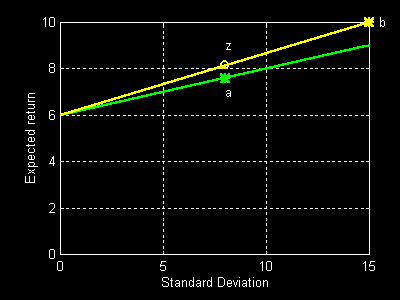For any desired level of risk, a portfolio "based on" asset b will provide a higher expected return than one based on asset a. In this context, the phrase "based on" connotes a combination of the asset in question and the riskless asset with the amount of the latter positive, negative or zero as required to obtain the desired level of risk. Thus if the level of risk offered by asset a is desired, the combination of asset b and lending that provides the risk and return shown by point z in the diagram should be selected, since it provides the same level of risk, but greater expected return. This will be true for any desired level of risk.

Another way to see this is to consider an investment of x1 in asset 1 and x2 in asset 2 as (1) an investment in asset 1 and (2) a decision to take a position of size x2 in the zero-investment strategy in which asset 1 is shorted, with the proceeds from the short sale invested in asset 2. An institutional arrangement for the latter is a swap in which the Investor (party A) agrees to pay a fixed rate (the return on asset 1) to a counterparty (party B) and the latter agrees to pay a rate based on the return of a risky asset (asset 2). Both rates are multiplied by a notional amount, then netted to determine the final value transferred from one party ("the winner") to the other ("the loser").

In this situation the Investor will receive r1 on his or her direct investment and x*(r1-r2) on the swap, where x is the ratio of the notional value of the swap to the value of the Investor's original funds. Overall, the return will be:

```
r1 + x*(r2-r1)
```
```or:
```
```
r1*(1-x) + x*r2
```

Thus x plays the role of x2 in our original formulation, while (1-x) plays the role of x1.

While x represents a natural measure for use when contracting for a zero-investment strategy, in many cases a more useful measure of scale is the standard deviation of ending value. In this case we normalize it, dividing by amount of the Investor's initial fund to obtain the resultant standard deviation of overall return:

```
sp = x*s2
```

We are now ready to re-examine the choice between two mutually exclusive risky assets under the  assumption that it is possible to take long or short positions as desired, either directly or indirectly via  derivative strategies such as swaps.  In the present view, one must select between two zero-investment  strategies.  One provides e(xra)/s(xra) per unit of risk, while the other provides e(xrb)/s(xrb) per unit of  risk.  Assume that a fixed amount of risk, sp, is desired.  Then the expected returns of the two strategies  will be:

```
ea = e1+ ((e(xra)/s(sra))*sp
```
```and
```
```
eb = e1+ ((e(xrb)/s(srb))*sp
```

Clearly the strategy with the larger ratio of expected excess return to standard deviation of excess return is better. But this ratio is the excess return Sharpe Ratio.  In a situation of this sort one should pick the  alternative that provides the highest reward per unit of variability.  More simply put: among mutually    exclusive risky portfolios, pick the one with the greatest expected return Sharpe Ratio.

Practitioners often compute excess return Sharpe Ratios based on historic returns for mutual funds and other investment products. The usefulness of such measures may be limited due to lack of conformance with the assumptions that we have made in this section. First, the distribution of historic returns may be a poor surrogate for the distribution of next period's return. Second, it may not be possible to borrow at the same rate of interest used in the calculations of excess return. Finally, the Investor may have other assets and/or liabilities, and the funds being compared may provide different degrees of correlation with them. Since the Sharpe Ratio takes into account only expected return and risk, it may fail to lead to the best investment if correlations with important assets and liabilities differ significantly among the alternatives.

Despite these caveats, the Sharpe Ratio is a useful measure that can combine aspects of both expected return and risk in one number. The excess return Sharpe Ratio represents one application of the broader concept. Later sections present other examples.